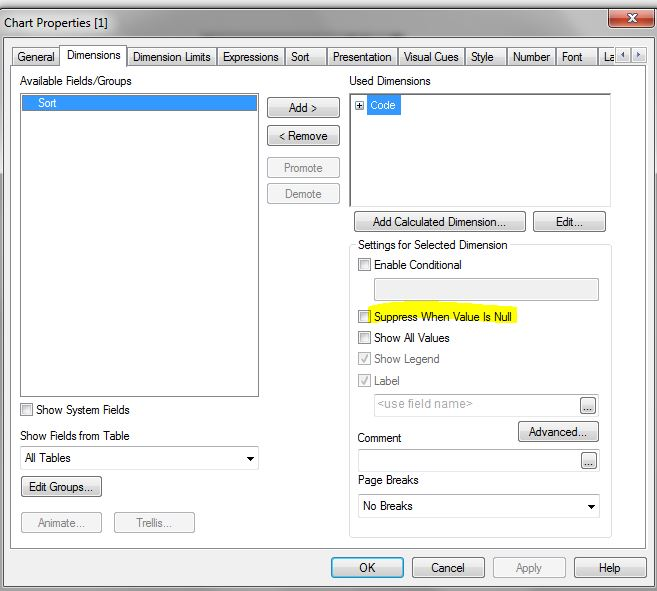# QlikView App Development

Discussion Board for collaboration related to QlikView App Development.

Honored Contributor II

## Show all columns in calculated dimension

I have a calculated dimension in a pivot table as below:

=Aggr(If(avg(Aggr(Sum(force)/2),Name,ID)) <=100,'Low',

If(avg(Aggr(Sum(force)/2),Name,ID)) > 100 And avg(Aggr(Sum(force)/2),Name,ID)) <=300,'Medium',

If(avg(Aggr(Sum(force)/2),Name,ID)) > 300,'High'))),Name,ID)

It is working fine and shows the columns as Low, Medium and High when I have values for each. If I have values only for Medium then it shows only "Medium" column.

The question is how can I show all the three columns all the time regardless if it has a value or not.

Example:

Wrong:

Low     Medium     High

20

Correct:    <--- This is how it should display

Low     Medium     High

0           20             0

Is this possible ?

Thanks

Tags (1)
27 Replies
Honored Contributor II

## Re: Show all columns in calculated dimension

Here is the updated example:

Example:

Wrong:

Medium

20

Correct:    <--- This is how it should display

Low     Medium     High

0           20             0

Thanks

Contributor II

## Re: Show all columns in calculated dimension

Hi,

Please make sure the below option is unchecked.

Dimensions -- Supress when value is Null.Honored Contributor III

## Re: Show all columns in calculated dimension

Check Show all values as well.MVP

## Re: Show all columns in calculated dimension

Probably not the "right" solution, and not even positive it would work, but how about using 0 as your null and missing symbols on the presentation tab o the chart?

Honored Contributor II

## Re: Show all columns in calculated dimension

No, It is not working.. Thanks

Honored Contributor II

## Re: Show all columns in calculated dimension

Honored Contributor II

## Re: Show all columns in calculated dimension

it is greyed out... Thanks

Honored Contributor III

## Re: Show all columns in calculated dimension

right, I realized it doesn't appear active for calculated dimension.

Can you share your expression? One confusing point is on one side we want to show dimension based on condition result and contradicting to that we want to show all dimensions.

I was thinking Valuelist as a synthetic dimension can help here bcz that way all constant dimensions can be shown all the time and measures can be calculated in expression using Pick(match(Valuelist().

Honored Contributor II

## Re: Show all columns in calculated dimension

Below are the three expressions I am using:

Exp1:

# of Trips  =Count(Name)

Exp2:

Used = Avg(Aggr(count({<Record={'XXX'}>} DISTINCT [Tripsused]),Name,ID))

Exp3:

Per_Day = avg(Aggr(Sum(force)/2),Name,ID))

Thanks